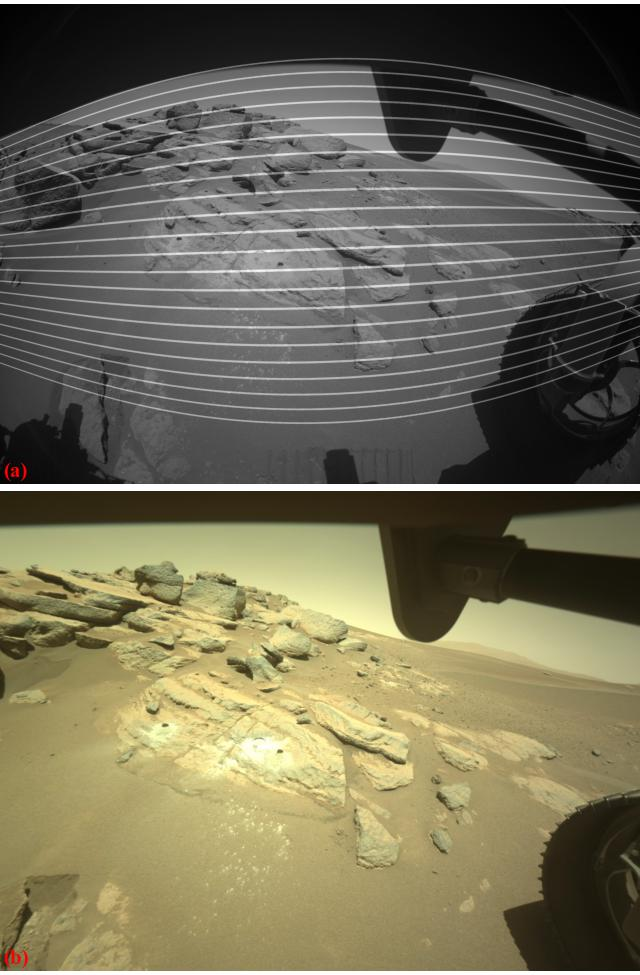# 3.3.8. Correct radial distortion of an image without using a calibration target

In the previous demos, distortion coefficients are determined by using a calibration target. In practice, we may have to correct radial distortion of an image but don’t have the calibration image taken from the same camera. The following workflow shows how to do that on images acquired by the front-right hazard-camera of the Percy Rover

• Load the image, create a line-pattern for visual inspection (Fig. 75). The idea is that we apply an estimated forward model to the line-pattern and overlay the result on top of the image.

```import os
import numpy as np
import discorpy.proc.processing as proc
import discorpy.post.postprocessing as post
import scipy.ndimage as ndi

file_path = "../../data/percy_cam/F_R_hazcam.png"
output_base = "E:/output_demo_08/"
mat0 = mat0 / np.max(mat0)
(height, width) = mat0.shape

# Create a line-pattern image
line_pattern = np.zeros((height, width), dtype=np.float32)
for i in range(50, height - 50, 40):
line_pattern[i - 2:i + 3] = 1.0

# Estimate parameters by visual inspection:
# Coarse estimation
xcenter = width // 2
ycenter = height // 2
list_power = np.asarray([1.0, 10**(-4), 10**(-7), 10**(-10), 10**(-13)])
list_coef = np.asarray([1.0, 1.0, 1.0, 1.0, 1.0])

# Rotate the line-pattern image if need to
angle = 2.0 # Degree
pad = width // 2 # Need padding as lines are shrunk after warping.
```Fig. 75 . (a) Image from the Percy Rover. (b) Generated line-pattern.
• The following codes show how to adjust parameters of a forward model, apply to the line-pattern, overlay to the Percy’s image, and make sure the warped lines are matching with the curve feature in the Percy’s image. If there are lines in the image which we can be certain that they are straight, an automated method can be developed to extract these lines and match with warped lines from the line-pattern. For the currently used image, we simply use visual inspection (Fig. 76).

```# Define scanning routines
def scan_coef(idx, start, stop, step, list_coef, list_power, output_base0, mat0,
output_base = output_base0 + "/coef_" + str(idx) + "_ntime_" + str(ntime)
while os.path.isdir(output_base):
ntime = ntime + 1
output_base = output_base0 + "/coef_" + str(idx) + "_ntime_" + str(ntime)
(height, width) = mat0.shape
for num in np.arange(start, stop + step, step):
list_coef_est = np.copy(list_coef)
list_coef_est[idx] = list_coef_est[idx] + num
list_ffact = list_power * list_coef_est
name = "coef_{0}_val_{1:4.2f}".format(idx, list_coef_est[idx])
io.save_image(output_base + "/forward/img_" + name + ".jpg", mat0 + 0.5 * line_img_warped)
if backward is True:
# Transform to the backward model for correction
hlines = np.int16(np.linspace(0, height, 40))
vlines = np.int16(np.linspace(0, width, 50))
ref_points = [[i - ycenter, j - xcenter] for i in hlines for j in vlines]
list_bfact = proc.transform_coef_backward_and_forward(list_ffact, ref_points=ref_points)
img_unwarped = post.unwarp_image_backward(mat0, xcenter, ycenter, list_bfact)
io.save_image(output_base + "/backward/img_" + name + ".jpg", img_unwarped)

def scan_center(xcenter, ycenter, start, stop, step, list_coef, list_power,
output_base = output_base0 + "/" + axis + "_center" + "_ntime_" + str(ntime)
while os.path.isdir(output_base):
ntime = ntime + 1
output_base = output_base0 + "/" + axis + "_center" + "_ntime_" + str(ntime)
(height, width) = mat0.shape
list_ffact = list_power * list_coef
if axis == "x":
for num in np.arange(start, stop + step, step):
list_ffact)
name = "xcenter_{0:7.2f}".format(xcenter + num)
io.save_image(output_base + "/forward/img_" + name + ".jpg", mat0 + 0.5 * line_img_warped)
if backward is True:
# Transform to the backward model for correction
hlines = np.int16(np.linspace(0, height, 40))
vlines = np.int16(np.linspace(0, width, 50))
ref_points = [[i - ycenter, j - xcenter] for i in hlines for j in vlines]
list_bfact = proc.transform_coef_backward_and_forward(list_ffact, ref_points=ref_points)
img_unwarped = post.unwarp_image_backward(mat0, xcenter+num, ycenter, list_bfact)
io.save_image(output_base + "/backward/img_" + name + ".jpg",  img_unwarped)
else:
for num in np.arange(start, stop + step, step):
list_ffact)
name = "ycenter_{0:7.2f}".format(ycenter + num)
io.save_image(output_base + "/forward/img_" + name + ".jpg", mat0 + 0.5 * line_img_warped)
if backward is True:
# Transform to the backward model for correction
hlines = np.int16(np.linspace(0, height, 40))
vlines = np.int16(np.linspace(0, width, 50))
ref_points = [[i - ycenter, j - xcenter] for i in hlines for j in vlines]
list_bfact = proc.transform_coef_backward_and_forward(list_ffact, ref_points=ref_points)
img_unwarped = post.unwarp_image_backward(mat0, xcenter, ycenter+num, list_bfact)
io.save_image(output_base + "/backward/img_" + name + ".jpg",  img_unwarped)

## Scan the 4th coefficient
## The value of 24.0 is good, update the 4th coefficient.
list_coef = 24.0

## Scan the 3rd coefficient
## The value of 2.0 is good, update the 3rd coefficient.
list_coef = 2.0

## Scan the 2nd coefficient
## The value of 5.0 is good, update the 2nd coefficient.
list_coef = 5.0

## Scan the x-center
scan_center(xcenter, ycenter, -50, 50, 2, list_coef, list_power, output_base,
## Found x=648 looks good.
xcenter = 646

## Scan the y-center
scan_center(xcenter, ycenter, -50, 50, 2, list_coef, list_power, output_base,
## Found y=480 looks good.
ycenter = 480
```
• The 0-order and 1st-order of polynomial coefficients control the scaling and the shearing of a warping image, we can adjust them to get the most of image staying inside the field-of-view. From the estimated coefficients of the forward model, it’s straightforward to calculate the coefficients of the backward model which is used to unwarp the Percy’s image.

```# Adjust the 1st-order and 0-order coefficients manually if need to.
list_coef = 1.0
list_coef = 1.0

# Get a good estimation of the forward model
list_ffact = list_coef * list_power
# Transform to the backward model for correction
ref_points = [[i - ycenter, j - xcenter] for i in range(0, height, 50) for j in
range(0, width, 50)]
list_bfact = proc.transform_coef_backward_and_forward(list_ffact, ref_points=ref_points)

img_corrected = np.copy(img)

# Unwarped each color channel of the image
for i in range(img.shape[-1]):
img_corrected[:, :, i] = post.unwarp_image_backward(img[:, :, i], xcenter,
ycenter, list_bfact)

# Save the unwarped image.
io.save_image(output_base + "/F_R_hazcam_unwarped.png", img_corrected)
```Fig. 76 . (a) Overlay between the warped line-pattern and the Percy’s image. (b) Unwarped image of Fig. 75 (a).
• From the determined coefficients, we can correct other images of the same camera (Fig. 77, Fig. 78).

```# Correct other images from the same camera:
for i in range(img.shape[-1]):
img_corrected[:, :, i] = post.unwarp_image_backward(img[:, :, i], xcenter,
ycenter, list_bfact)
io.save_image(output_base + "/rock_core1_unwarped.png", img_corrected)

Click `here` to download the Python codes.